What gravitational force does the moon produce on the Earth is their centers are 3.88×108 m apart and the moon has a mass of 7.3

Question

What gravitational force does the moon produce
on the Earth is their centers are 3.88×108 m apart
and the moon has a mass of 7.34×1022 kg?

in progress 0
5 months 2021-08-09T15:17:53+00:00 1 Answers 8 views 0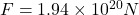Explanation:

Mass of earth ;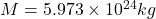Mass of moon ;distance between them is ;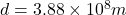Gravitational constant is given by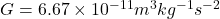So , the gravitational force between them is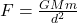Insert the values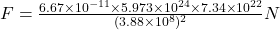So,Thus , the force between earth and moon is